# RD Sharma Solutions for Class 9 Maths Chapter 5 Factorization of Algebraic Expressions

## RD Sharma Solutions for Class 9 Maths Chapter 5 – Free PDF Download

RD Sharma Solutions for Class 9 Maths Chapter 5 – Factorization of Algebraic Expressions are given here in detail. This study material includes five exercises and all the questions are solved by BYJU’S subject experts. RD Sharma Class 9 Solutions Maths helps students to build the basics and in-depth understanding of the fundamental concepts. In this Chapter, students will learn about Factorization of Algebraic Expressions using the factorization method. The process of factorization can be defined as the disintegration of a term into smaller factors whereas the algebraic expressions are built up of variables, integer constants, and basic arithmetic operations of algebra.

The expert faculty at BYJU’S have formulated these RD Sharma Solutions based on the marks allotted for each step of a problem. The main objective is to help students in solving problems effortlessly, in turn, which makes the subject easier to understand. Our experts have provided chapter-wise solutions in a precise manner which boosts students academic performance. Students can download the RD Sharma Solutions  to procure high marks in the examination.

## Download PDF of RD Sharma Solutions for Class 9 Maths Chapter 5 Factorization of Algebraic Expressions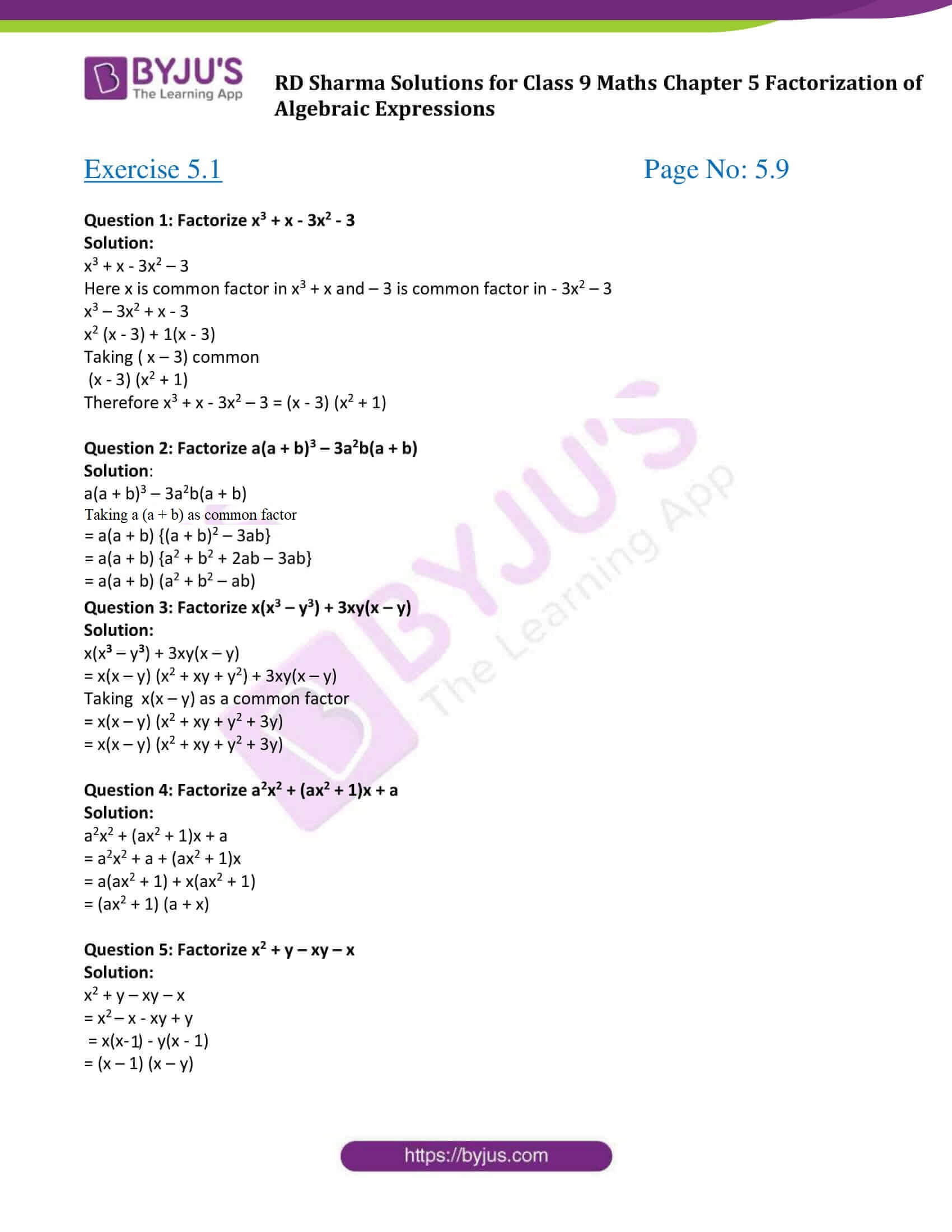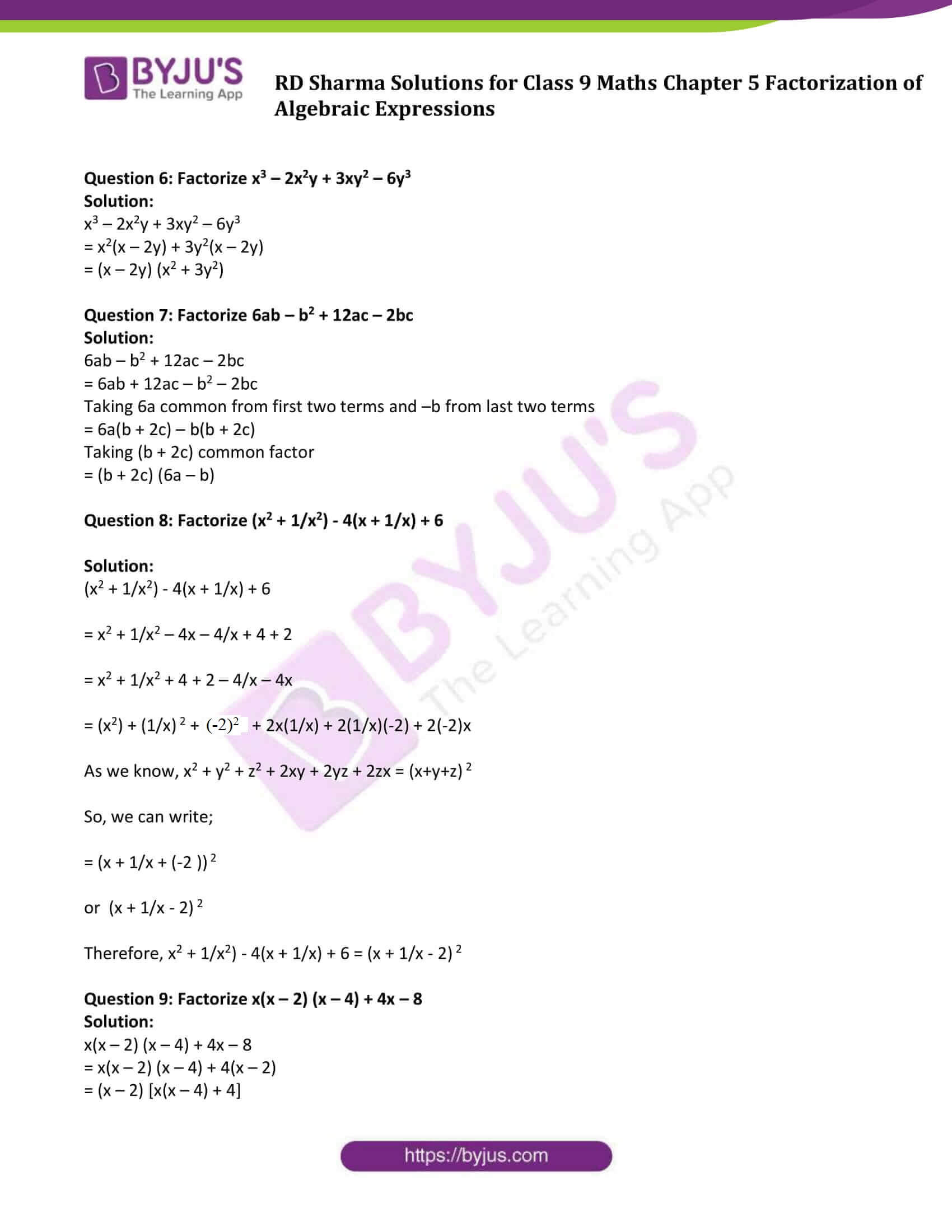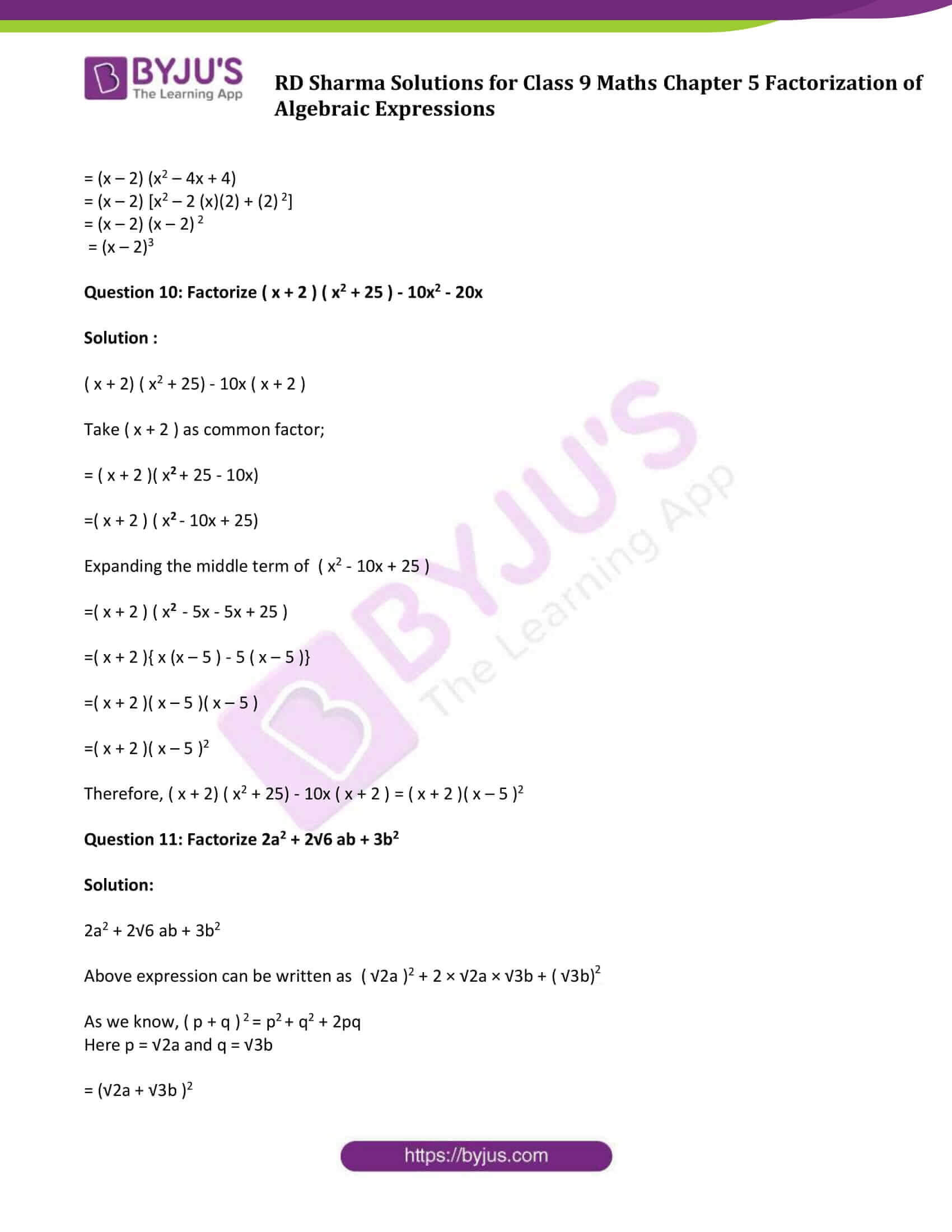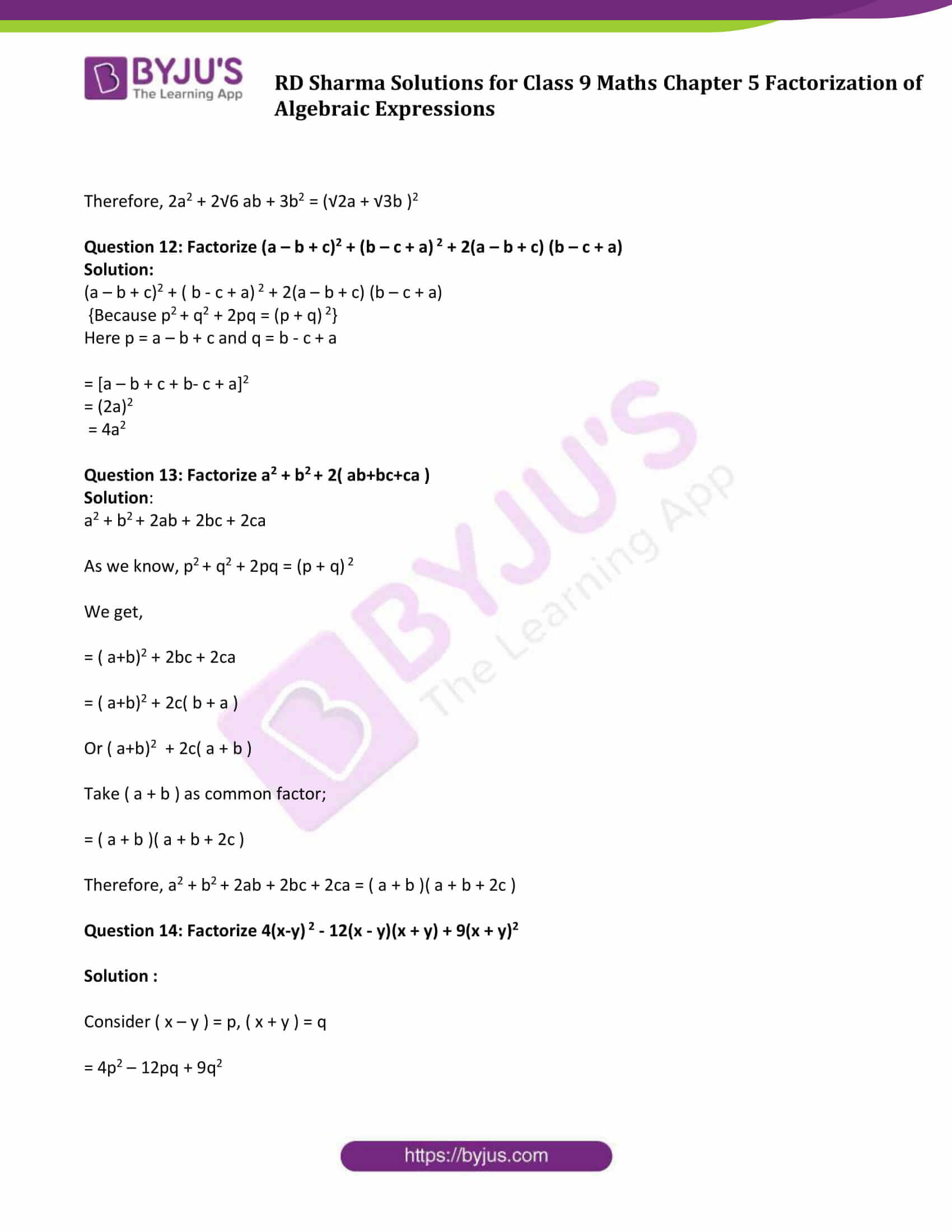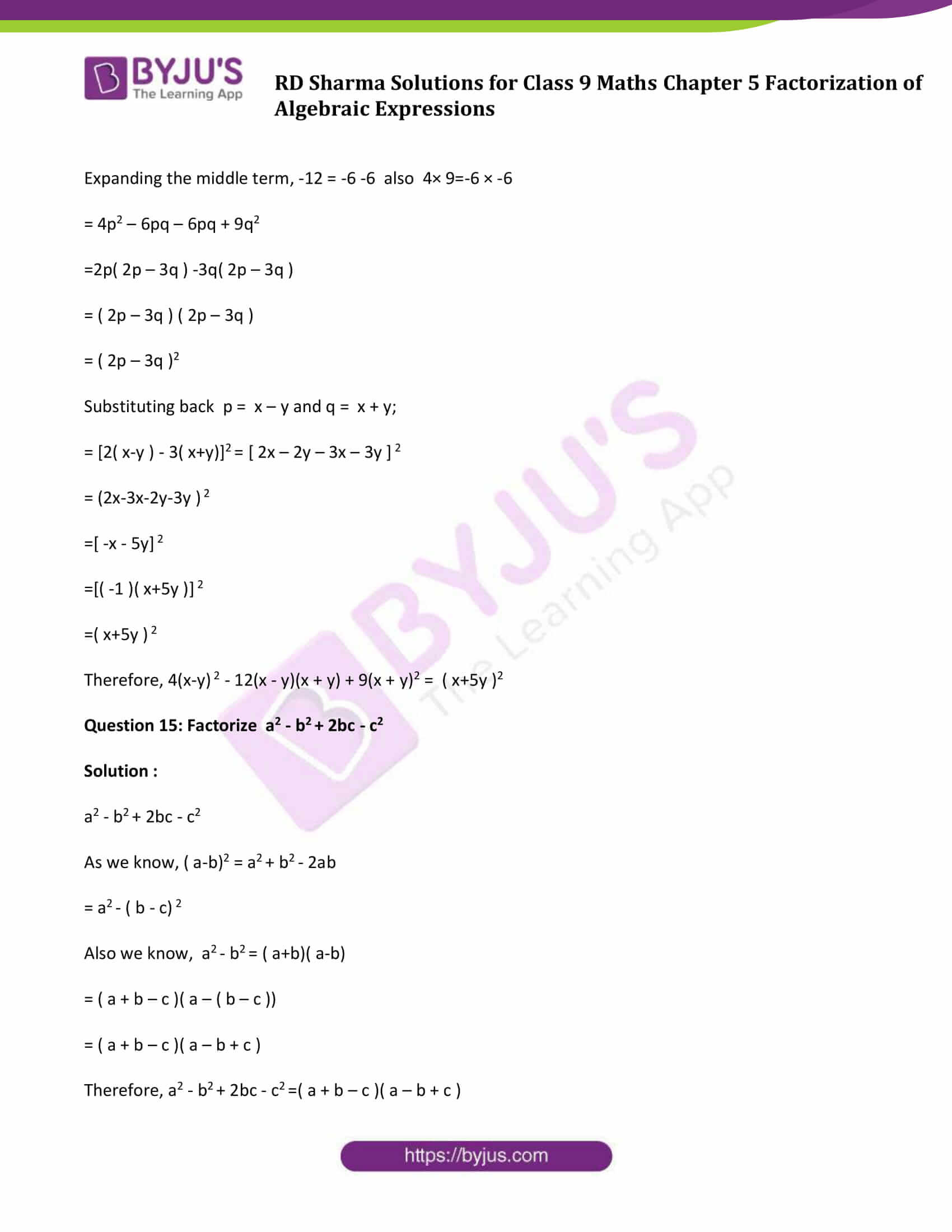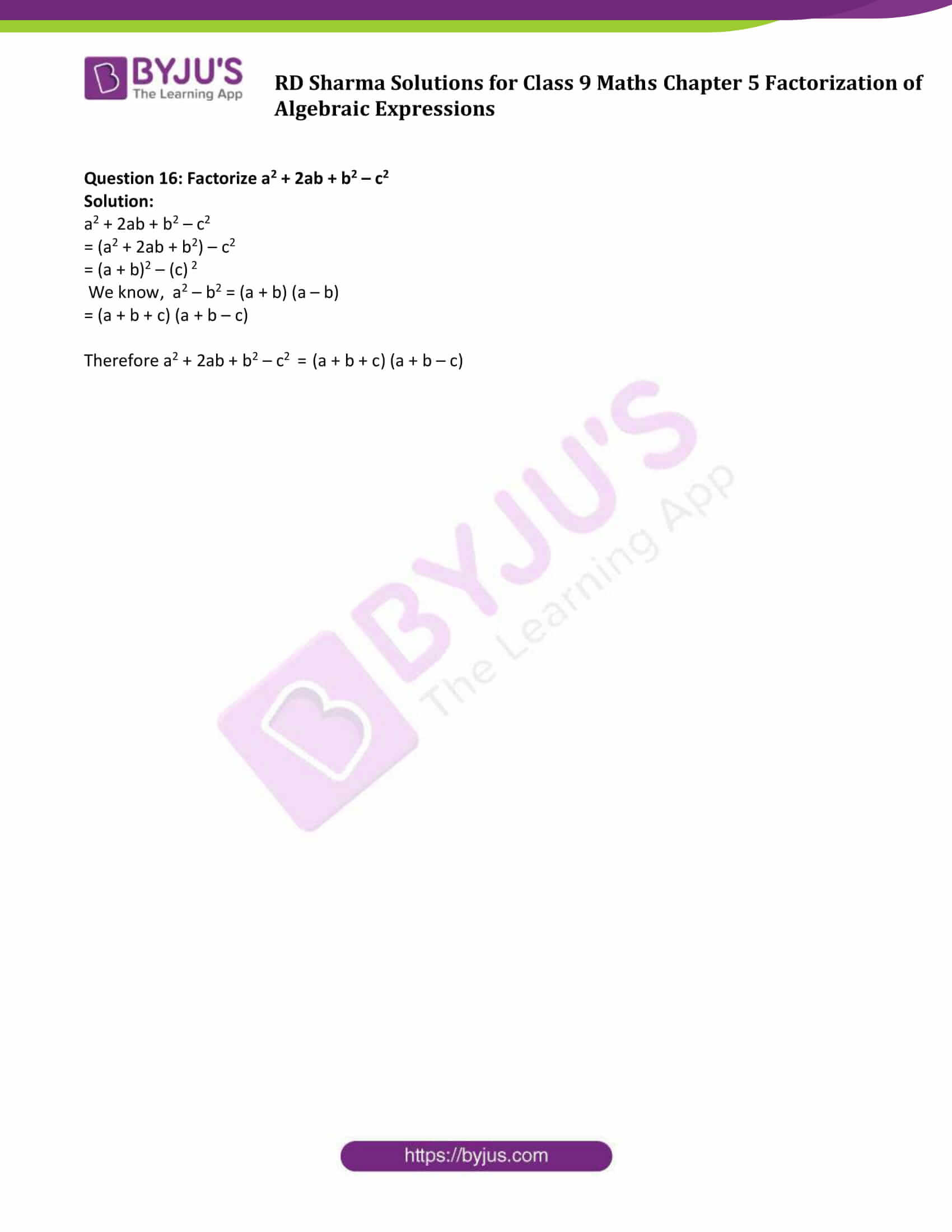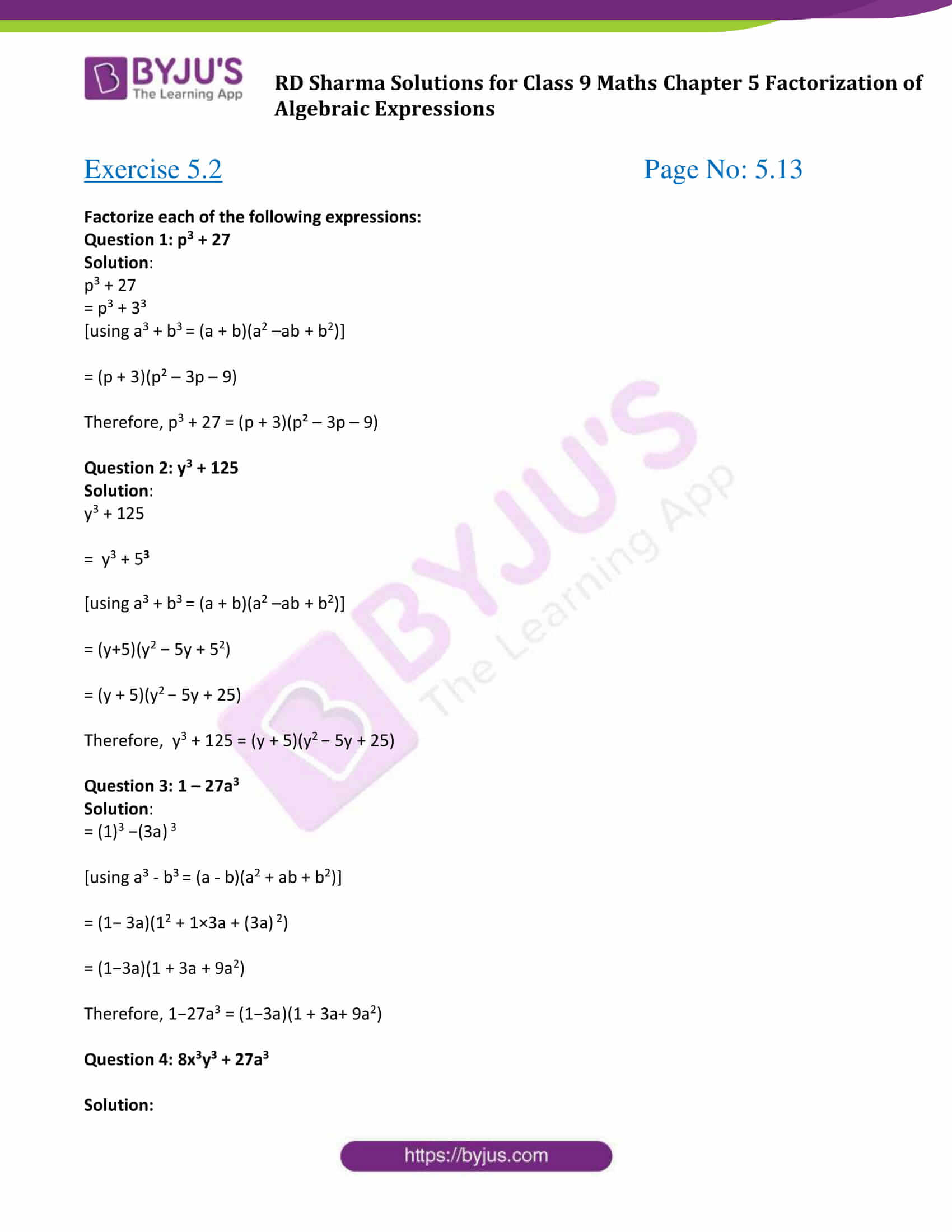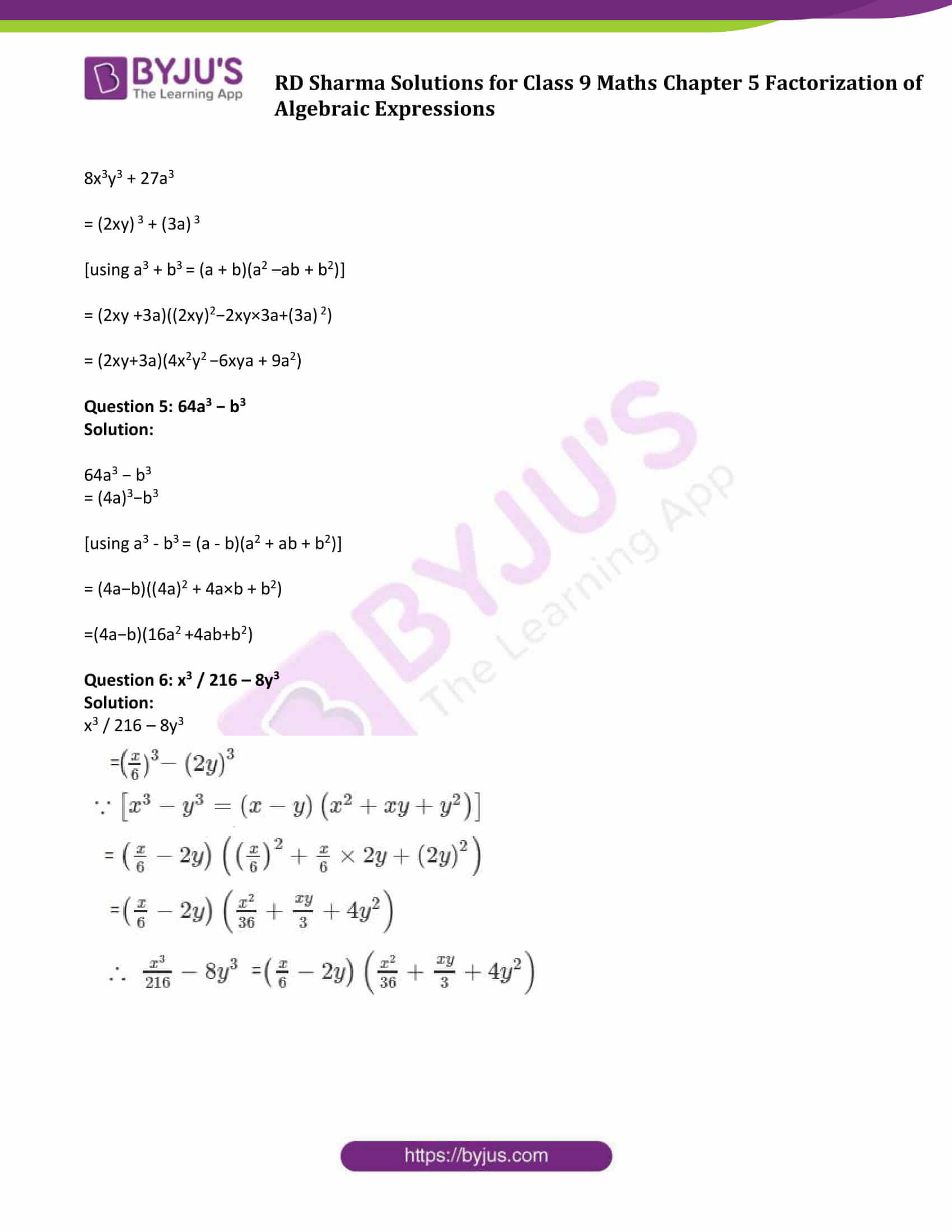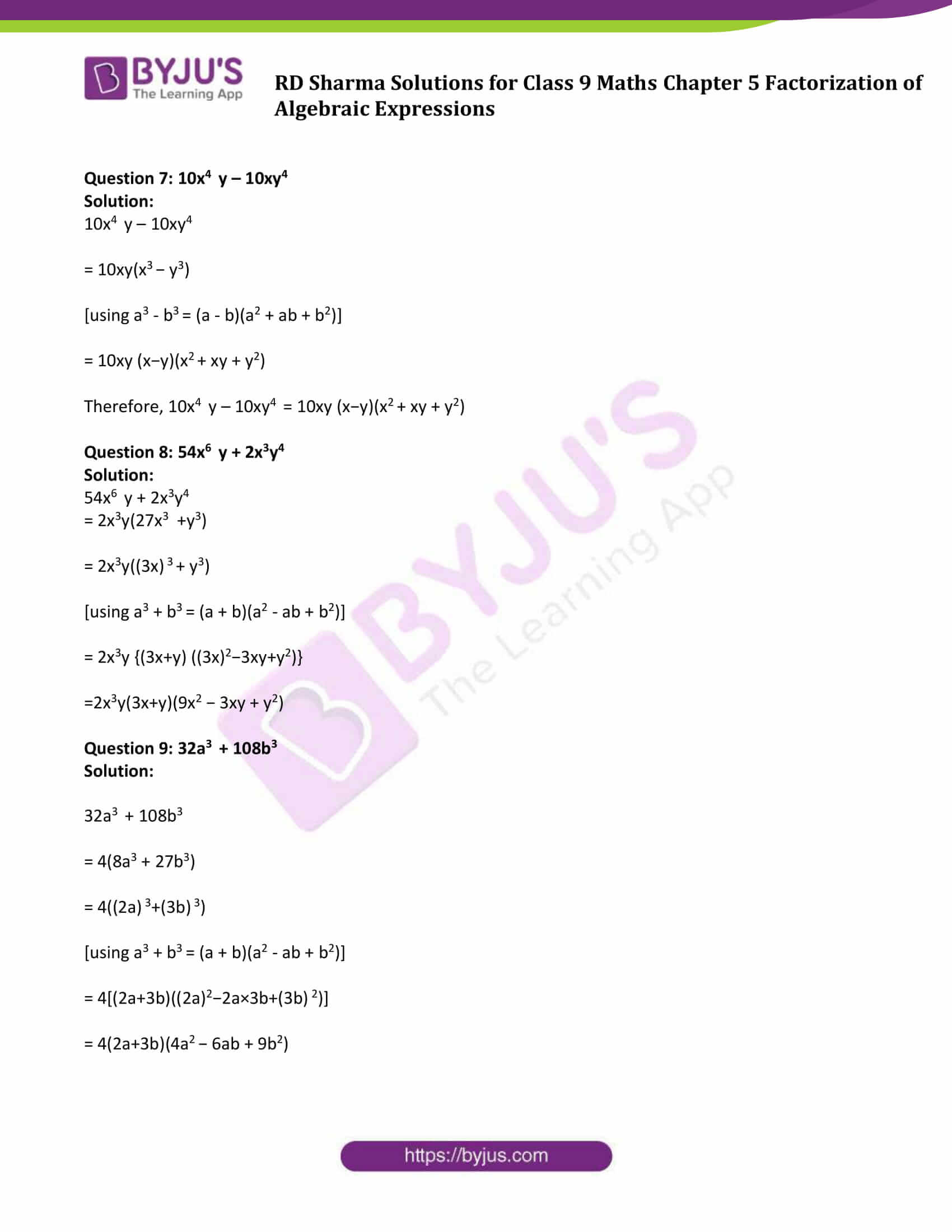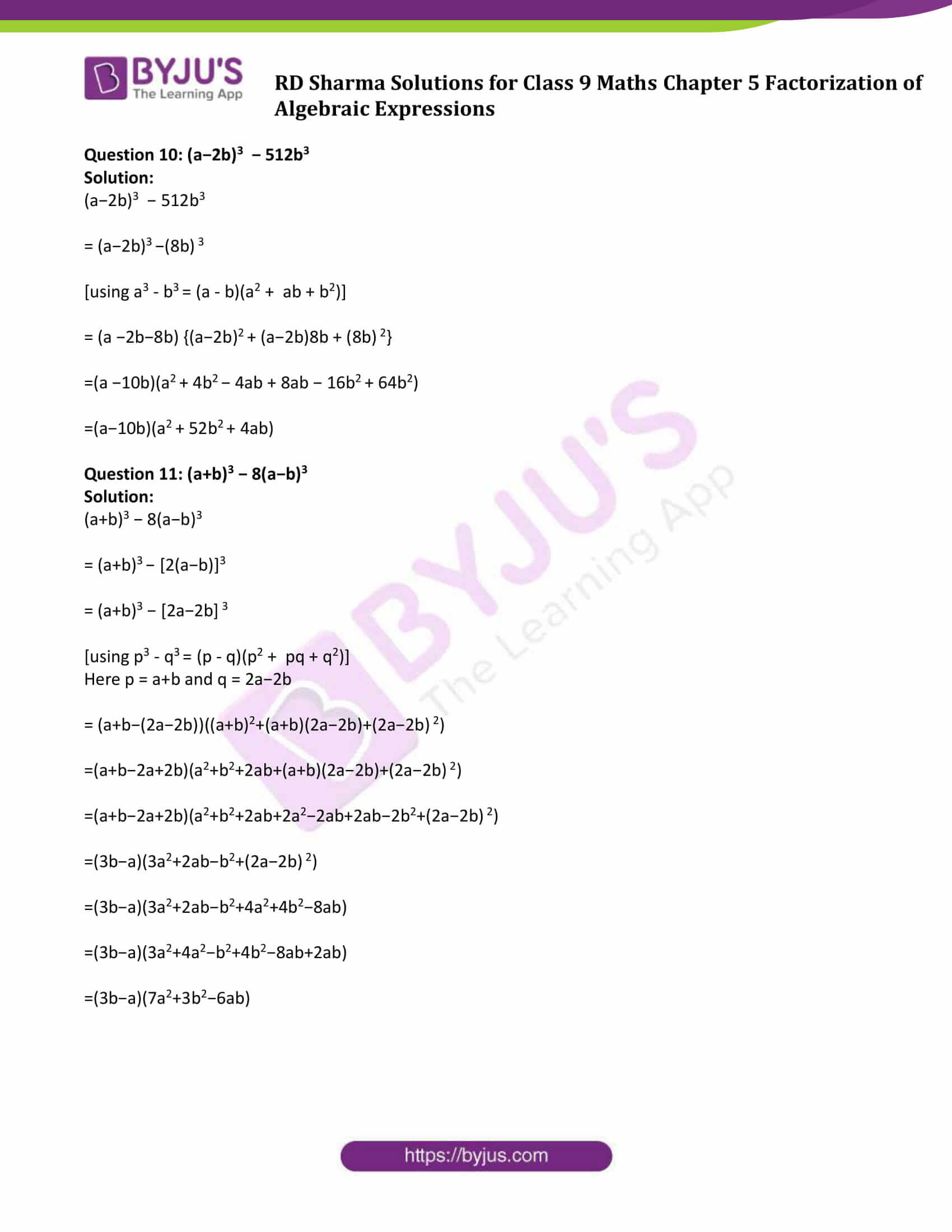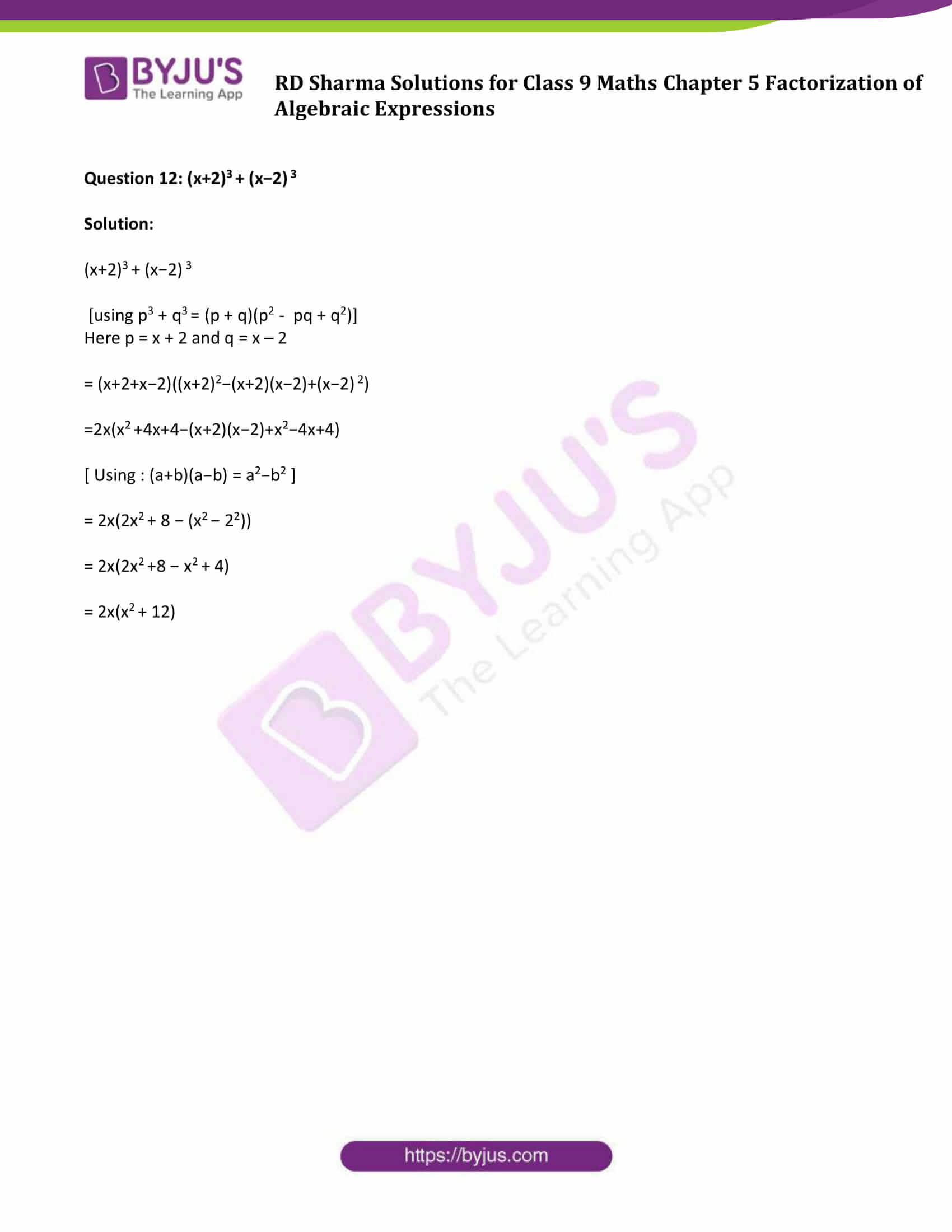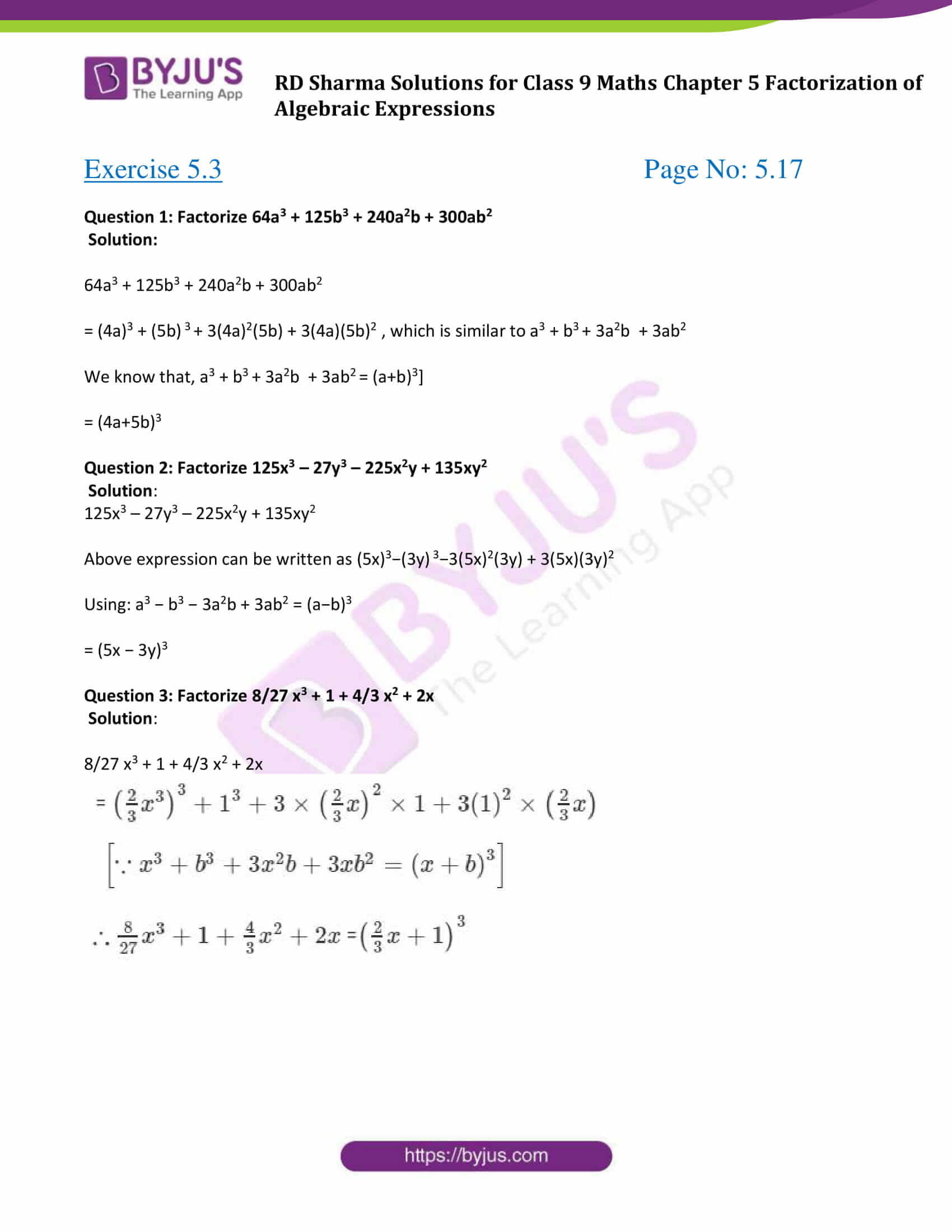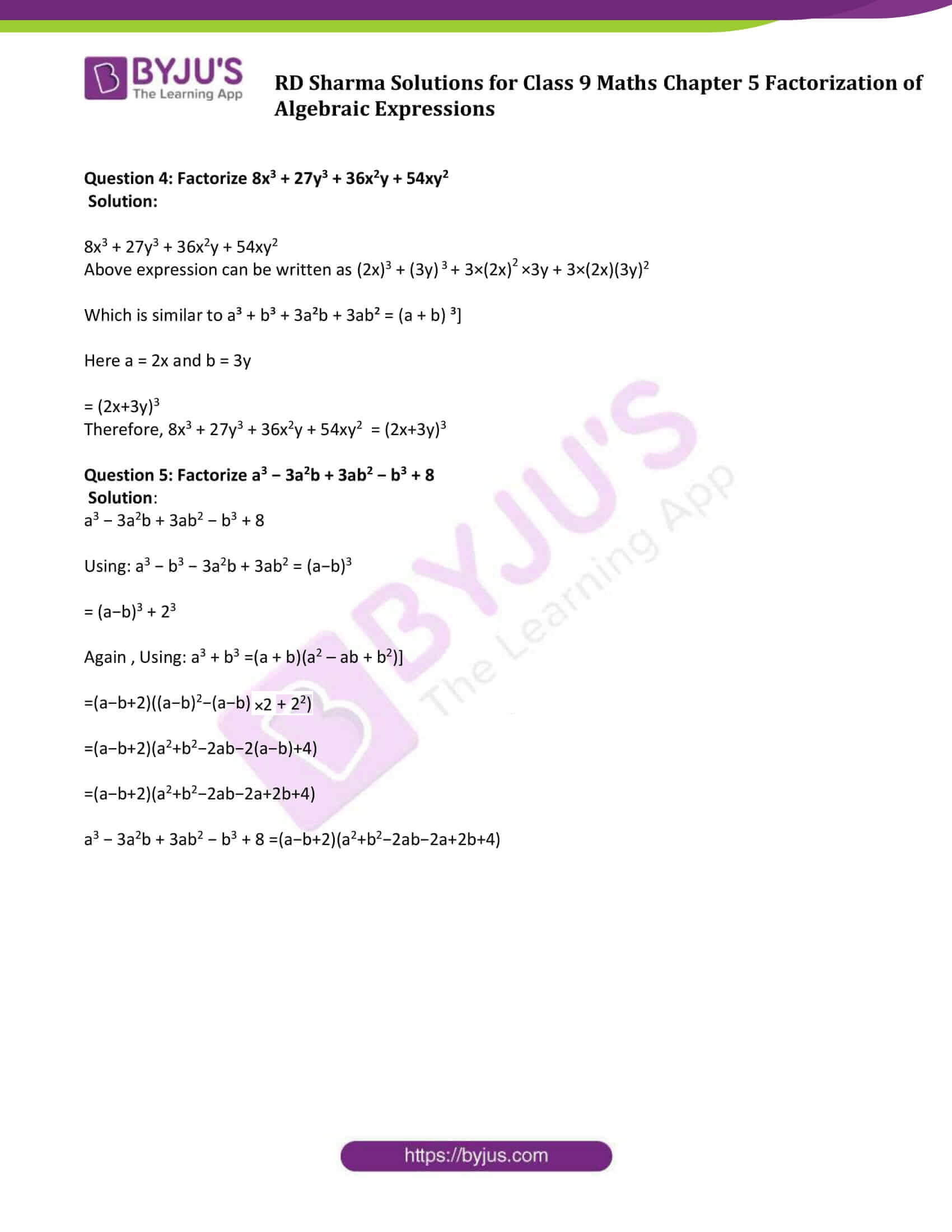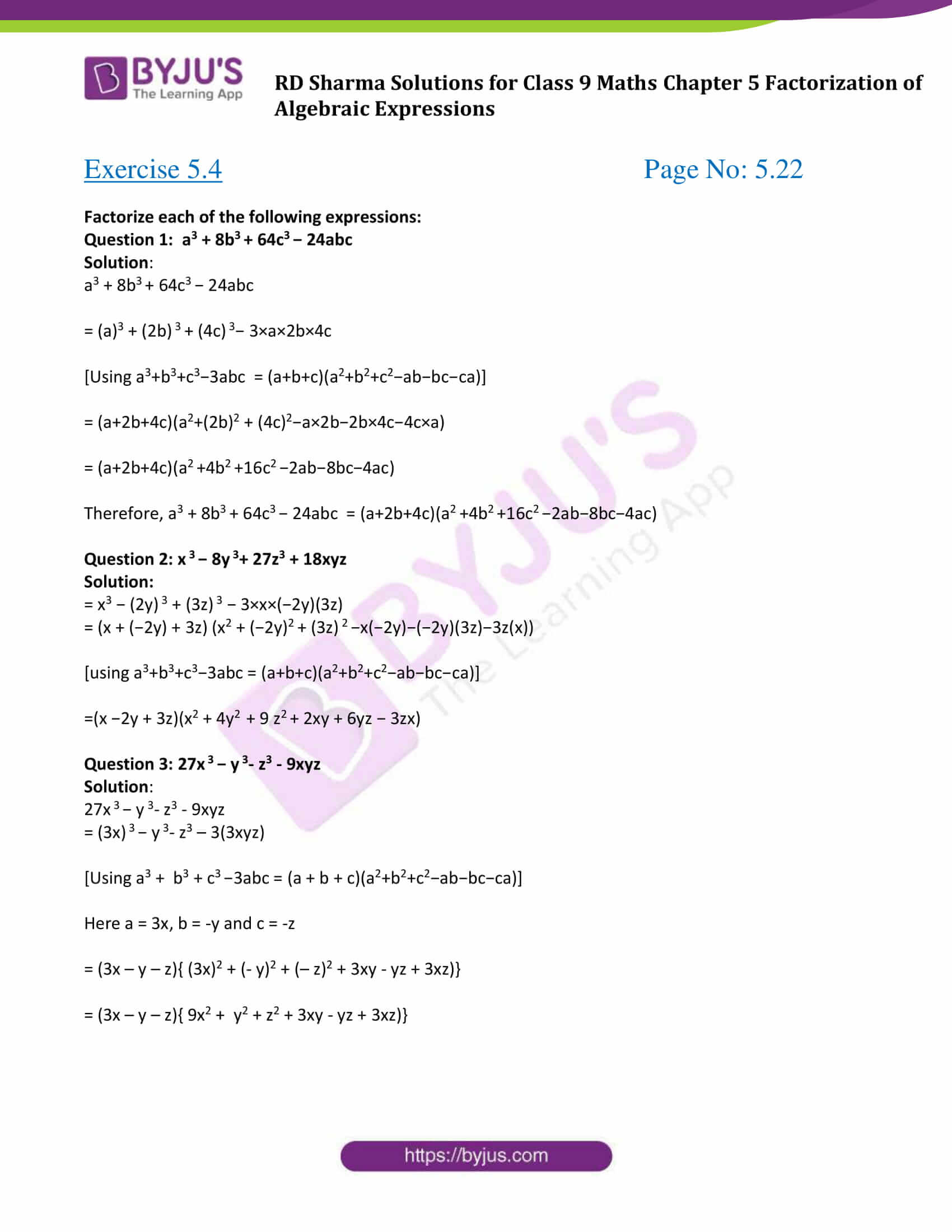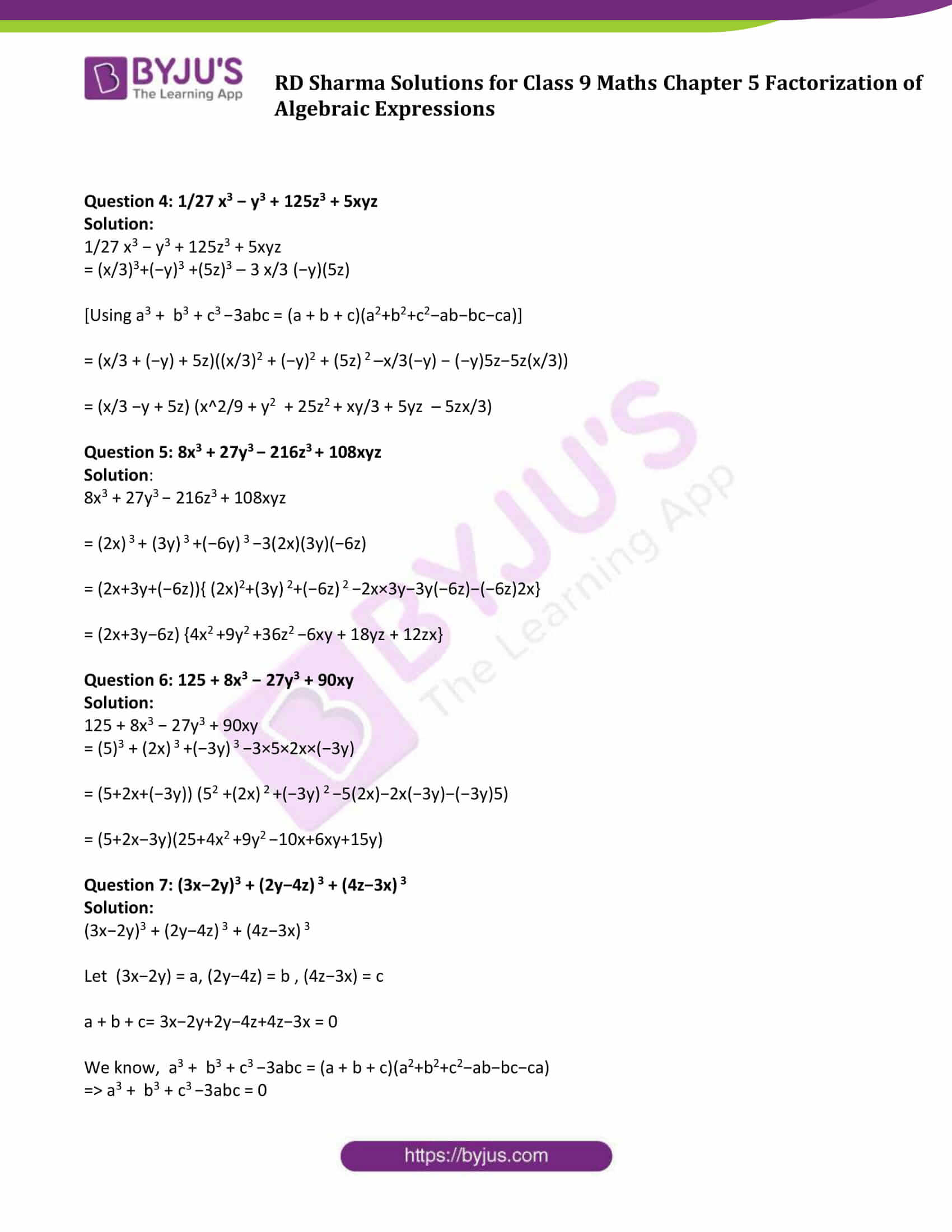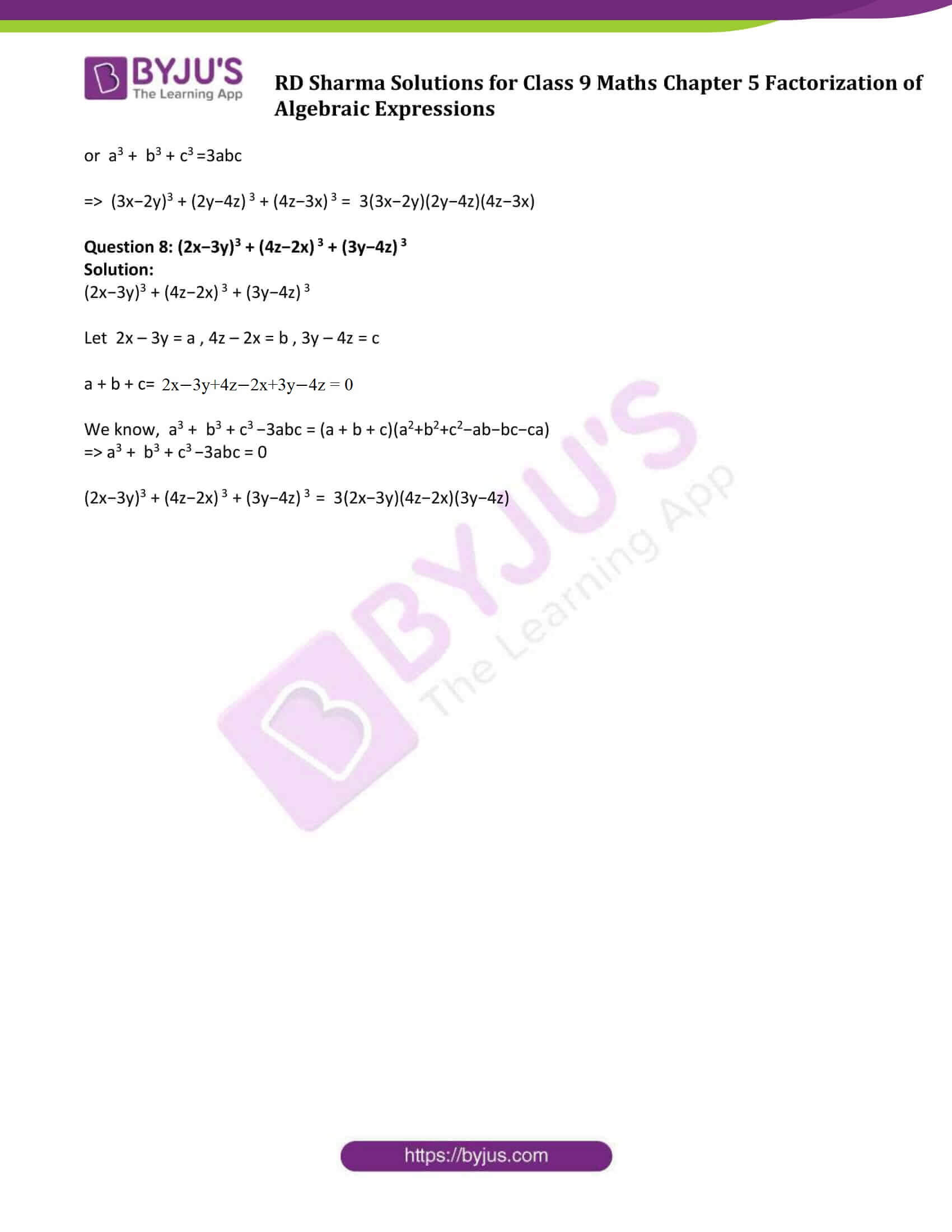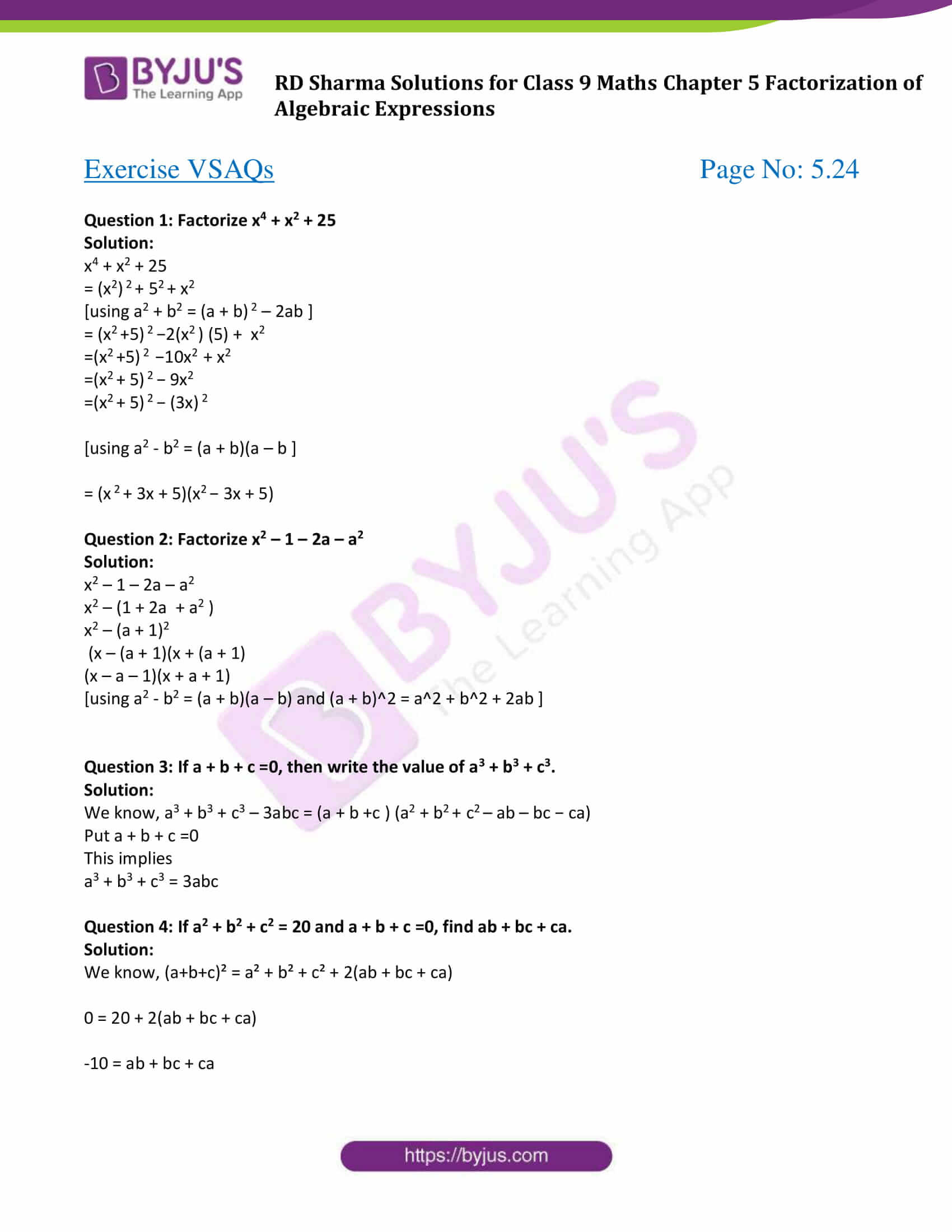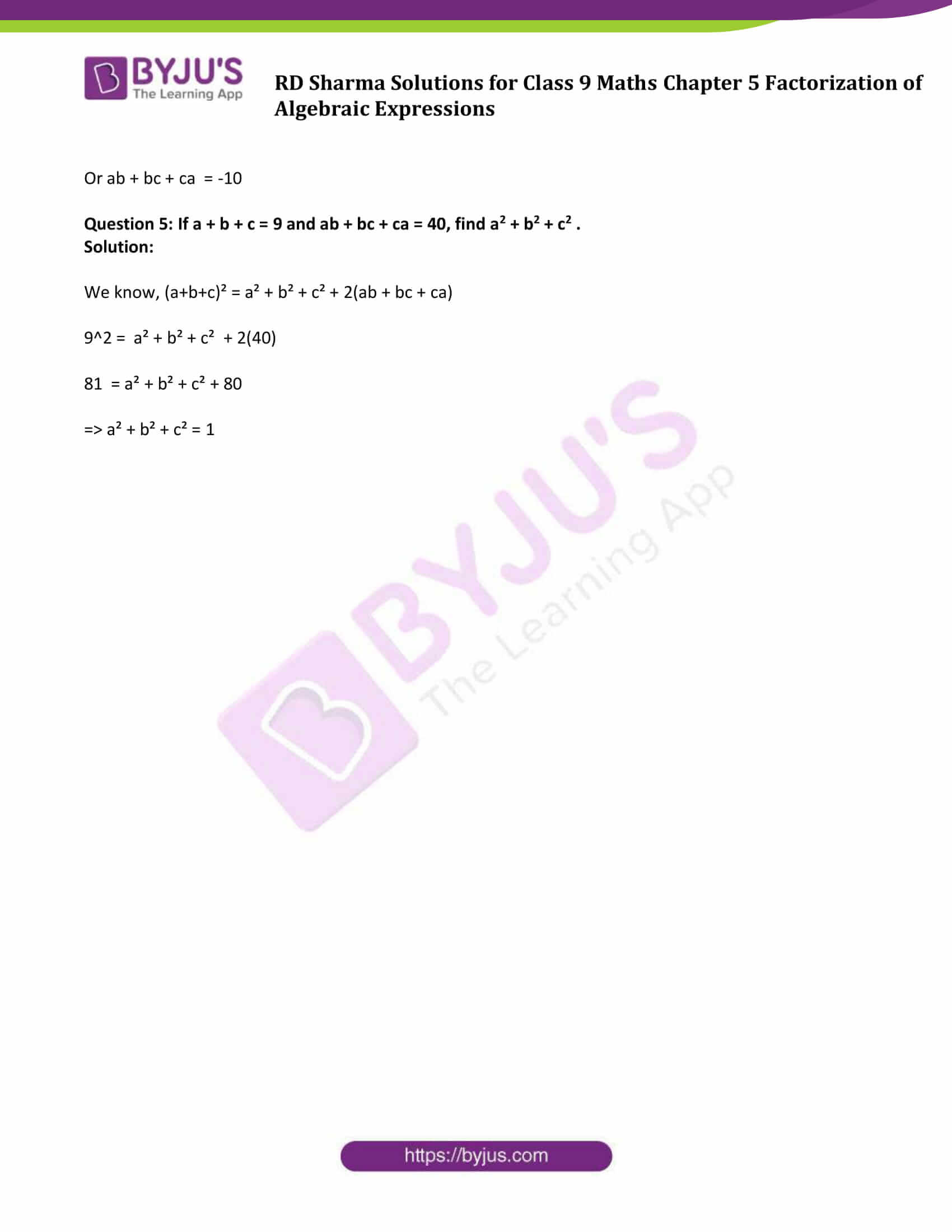### Exercise 5.1 Page No: 5.9

Question 1: Factorize x3 + x – 3x2 – 3

Solution:

x3 + x – 3x2 – 3

Here x is common factor in x3 + x and – 3 is common factor in – 3x2 – 3

x3 – 3x2 + x – 3

x2 (x – 3) + 1(x – 3)

Taking ( x – 3) common

(x – 3) (x2 + 1)

Therefore x3 + x – 3x2 – 3 = (x – 3) (x2 + 1)

Question 2: Factorize a(a + b)3 – 3a2b(a + b)

Solution:

a(a + b)3 – 3a2b(a + b)

Taking a (a + b) as common factor

= a(a + b) {(a + b)2 – 3ab}

= a(a + b) {a2 + b2 + 2ab – 3ab}

= a(a + b) (a2 + b2 – ab)

Question 3: Factorize x(x3 – y3) + 3xy(x – y)

Solution:

x(x3 – y3) + 3xy(x – y)

= x(x – y) (x2 + xy + y2) + 3xy(x – y)

Taking x(x – y) as a common factor

= x(x – y) (x2 + xy + y2 + 3y)

= x(x – y) (x2 + xy + y2 + 3y)

Question 4: Factorize a2x2 + (ax2 + 1)x + a

Solution:

a2x2 + (ax2 + 1)x + a

= a2x2 + a + (ax2 + 1)x

= a(ax2 + 1) + x(ax2 + 1)

= (ax2 + 1) (a + x)

Question 5: Factorize x2 + y – xy – x

Solution:

x2 + y – xy – x

= x2 – x – xy + y

= x(x- 1) – y(x – 1)

= (x – 1) (x – y)

Question 6: Factorize x3 – 2x2y + 3xy2 – 6y3

Solution:

x3 – 2x2y + 3xy2 – 6y3

= x2(x – 2y) + 3y2(x – 2y)

= (x – 2y) (x2 + 3y2)

Question 7: Factorize 6ab – b2 + 12ac – 2bc

Solution:

6ab – b2 + 12ac – 2bc

= 6ab + 12ac – b2 – 2bc

Taking 6a common from first two terms and –b from last two terms

= 6a(b + 2c) – b(b + 2c)

Taking (b + 2c) common factor

= (b + 2c) (6a – b)

Question 8: Factorize (x2 + 1/x2) – 4(x + 1/x) + 6

Solution:

(x2 + 1/x2) – 4(x + 1/x) + 6

= x2 + 1/x2 – 4x – 4/x + 4 + 2

= x2 + 1/x2 + 4 + 2 – 4/x – 4x

= (x2) + (1/x) 2 + ( -2 )2 + 2x(1/x) + 2(1/x)(-2) + 2(-2)x

As we know, x2 + y2 + z2 + 2xy + 2yz + 2zx = (x+y+z) 2

So, we can write;

= (x + 1/x + (-2 )) 2

or (x + 1/x – 2) 2

Therefore, x2 + 1/x2) – 4(x + 1/x) + 6 = (x + 1/x – 2) 2

Question 9: Factorize x(x – 2) (x – 4) + 4x – 8

Solution:

x(x – 2) (x – 4) + 4x – 8

= x(x – 2) (x – 4) + 4(x – 2)

= (x – 2) [x(x – 4) + 4]

= (x – 2) (x2 – 4x + 4)

= (x – 2) [x2 – 2 (x)(2) + (2) 2]

= (x – 2) (x – 2) 2

= (x – 2)3

Question 10: Factorize ( x + 2 ) ( x2 + 25 ) – 10x2 – 20x

Solution :

( x + 2) ( x2 + 25) – 10x ( x + 2 )

Take ( x + 2 ) as common factor;

= ( x + 2 )( x2 + 25 – 10x)

=( x + 2 ) ( x2 – 10x + 25)

Expanding the middle term of ( x2 – 10x + 25 )

=( x + 2 ) ( x2 – 5x – 5x + 25 )

=( x + 2 ){ x (x – 5 ) – 5 ( x – 5 )}

=( x + 2 )( x – 5 )( x – 5 )

=( x + 2 )( x – 5 )2

Therefore, ( x + 2) ( x2 + 25) – 10x ( x + 2 ) = ( x + 2 )( x – 5 )2

Question 11: Factorize 2a2 + 2√6 ab + 3b2

Solution:

2a2 + 2√6 ab + 3b2

Above expression can be written as ( √2a )2 + 2 × √2a × √3b + ( √3b)2

As we know, ( p + q ) 2 = p2 + q2 + 2pq

Here p = √2a and q = √3b

= (√2a + √3b )2

Therefore, 2a2 + 2√6 ab + 3b2 = (√2a + √3b )2

Question 12: Factorize (a – b + c)2 + (b – c + a) 2 + 2(a – b + c) (b – c + a)

Solution:

(a – b + c)2 + ( b – c + a) 2 + 2(a – b + c) (b – c + a)

{Because p2 + q2 + 2pq = (p + q) 2}

Here p = a – b + c and q = b – c + a

= [a – b + c + b- c + a]2

= (2a)2

= 4a2

Question 13: Factorize a2 + b2 + 2( ab+bc+ca )

Solution:

a2 + b2 + 2ab + 2bc + 2ca

As we know, p2 + q2 + 2pq = (p + q) 2

We get,

= ( a+b)2 + 2bc + 2ca

= ( a+b)2 + 2c( b + a )

Or ( a+b)2 + 2c( a + b )

Take ( a + b ) as common factor;

= ( a + b )( a + b + 2c )

Therefore, a2 + b2 + 2ab + 2bc + 2ca = ( a + b )( a + b + 2c )

Question 14: Factorize 4(x-y) 2 – 12(x – y)(x + y) + 9(x + y)2

Solution :

Consider ( x – y ) = p, ( x + y ) = q

= 4p2 – 12pq + 9q2

Expanding the middle term, -12 = -6 -6 also 4× 9=-6 × -6

= 4p2 – 6pq – 6pq + 9q2

=2p( 2p – 3q ) -3q( 2p – 3q )

= ( 2p – 3q ) ( 2p – 3q )

= ( 2p – 3q )2

Substituting back p = x – y and q = x + y;

= [2( x-y ) – 3( x+y)]2 = [ 2x – 2y – 3x – 3y ] 2

= (2x-3x-2y-3y ) 2

=[ -x – 5y] 2

=[( -1 )( x+5y )] 2

=( x+5y ) 2

Therefore, 4(x-y) 2 – 12(x – y)(x + y) + 9(x + y)2 = ( x+5y )2

Question 15: Factorize a2 – b2 + 2bc – c2

Solution :

a2 – b2 + 2bc – c2

As we know, ( a-b)2 = a2 + b2 – 2ab

= a2 – ( b – c) 2

Also we know, a2 – b2 = ( a+b)( a-b)

= ( a + b – c )( a – ( b – c ))

= ( a + b – c )( a – b + c )

Therefore, a2 – b2 + 2bc – c2 =( a + b – c )( a – b + c )

Question 16: Factorize a2 + 2ab + b2 – c2

Solution:

a2 + 2ab + b2 – c2

= (a2 + 2ab + b2) – c2

= (a + b)2 – (c) 2

We know, a2 – b2 = (a + b) (a – b)

= (a + b + c) (a + b – c)

Therefore a2 + 2ab + b2 – c2 = (a + b + c) (a + b – c)

### Exercise 5.2 Page No: 5.13

Factorize each of the following expressions:

Question 1: p3 + 27

Solution:

p3 + 27

= p3 + 33

[using a3 + b3 = (a + b)(a2 –ab + b2)]

= (p + 3)(p² – 3p – 9)

Therefore, p3 + 27 = (p + 3)(p² – 3p – 9)

Question 2: y3 + 125

Solution:

y3 + 125

= y3 + 53

[using a3 + b3 = (a + b)(a2 –ab + b2)]

= (y+5)(y2 − 5y + 52)

= (y + 5)(y2 − 5y + 25)

Therefore, y3 + 125 = (y + 5)(y2 − 5y + 25)

Question 3: 1 – 27a3

Solution:

= (1)3 −(3a) 3

[using a3 – b3 = (a – b)(a2 + ab + b2)]

= (1− 3a)(12 + 1×3a + (3a) 2)

= (1−3a)(1 + 3a + 9a2)

Therefore, 1−27a3 = (1−3a)(1 + 3a+ 9a2)

Question 4: 8x3y3 + 27a3

Solution:

8x3y3 + 27a3

= (2xy) 3 + (3a) 3

[using a3 + b3 = (a + b)(a2 –ab + b2)]

= (2xy +3a)((2xy)2−2xy×3a+(3a) 2)

= (2xy+3a)(4x2y2 −6xya + 9a2)

Question 5: 64a3 − b3

Solution:

64a3 − b3

= (4a)3−b3

[using a3 – b3 = (a – b)(a2 + ab + b2)]

= (4a−b)((4a)2 + 4a×b + b2)

=(4a−b)(16a2 +4ab+b2)

Question 6: x3 / 216 – 8y3

Solution:

x3 / 216 – 8y3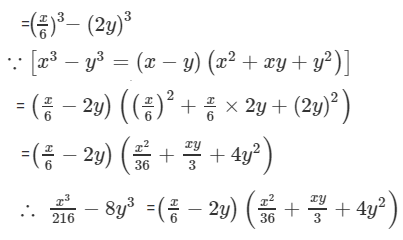Question 7: 10x4 y – 10xy4

Solution:

10x4 y – 10xy4

= 10xy(x3 − y3)

[using a3 – b3 = (a – b)(a2 + ab + b2)]

= 10xy (x−y)(x2 + xy + y2)

Therefore, 10x4 y – 10xy4 = 10xy (x−y)(x2 + xy + y2)

Question 8: 54x6 y + 2x3y4

Solution:

54x6 y + 2x3y4

= 2x3y(27x3 +y3)

= 2x3y((3x) 3 + y3)

[using a3 + b3 = (a + b)(a2 – ab + b2)]

= 2x3y {(3x+y) ((3x)2−3xy+y2)}

=2x3y(3x+y)(9x2 − 3xy + y2)

Question 9: 32a3 + 108b3

Solution:

32a3 + 108b3

= 4(8a3 + 27b3)

= 4((2a) 3+(3b) 3)

[using a3 + b3 = (a + b)(a2 – ab + b2)]

= 4[(2a+3b)((2a)2−2a×3b+(3b) 2)]

= 4(2a+3b)(4a2 − 6ab + 9b2)

Question 10: (a−2b)3 − 512b3

Solution:

(a−2b)3 − 512b3

= (a−2b)3 −(8b) 3

[using a3 – b3 = (a – b)(a2 + ab + b2)]

= (a −2b−8b) {(a−2b)2 + (a−2b)8b + (8b) 2}

=(a −10b)(a2 + 4b2 − 4ab + 8ab − 16b2 + 64b2)

=(a−10b)(a2 + 52b2 + 4ab)

Question 11: (a+b)3 − 8(a−b)3

Solution:

(a+b)3 − 8(a−b)3

= (a+b)3 − [2(a−b)]3

= (a+b)3 − [2a−2b] 3

[using p3 – q3 = (p – q)(p2 + pq + q2)]

Here p = a+b and q = 2a−2b

= (a+b−(2a−2b))((a+b)2+(a+b)(2a−2b)+(2a−2b) 2)

=(a+b−2a+2b)(a2+b2+2ab+(a+b)(2a−2b)+(2a−2b) 2)

=(a+b−2a+2b)(a2+b2+2ab+2a2−2ab+2ab−2b2+(2a−2b) 2)

=(3b−a)(3a2+2ab−b2+(2a−2b) 2)

=(3b−a)(3a2+2ab−b2+4a2+4b2−8ab)

=(3b−a)(3a2+4a2−b2+4b2−8ab+2ab)

=(3b−a)(7a2+3b2−6ab)

Question 12: (x+2)3 + (x−2) 3

Solution:

(x+2)3 + (x−2) 3

[using p3 + q3 = (p + q)(p2 – pq + q2)]

Here p = x + 2 and q = x – 2

= (x+2+x−2)((x+2)2−(x+2)(x−2)+(x−2) 2)

=2x(x2 +4x+4−(x+2)(x−2)+x2−4x+4)

[ Using : (a+b)(a−b) = a2−b2 ]

= 2x(2x2 + 8 − (x2 − 22))

= 2x(2x2 +8 − x2 + 4)

= 2x(x2 + 12)

### Exercise 5.3 Page No: 5.17

Question 1: Factorize 64a3 + 125b3 + 240a2b + 300ab2

Solution:

64a3 + 125b3 + 240a2b + 300ab2

= (4a)3 + (5b) 3 + 3(4a)2(5b) + 3(4a)(5b)2 , which is similar to a3 + b3 + 3a2b + 3ab2

We know that, a3 + b3 + 3a2b + 3ab2 = (a+b)3]

= (4a+5b)3

Question 2: Factorize 125x3 – 27y3 – 225x2y + 135xy2

Solution:

125x3 – 27y3 – 225x2y + 135xy2

Above expression can be written as (5x)3−(3y) 3−3(5x)2(3y) + 3(5x)(3y)2

Using: a3 − b3 − 3a2b + 3ab2 = (a−b)3

= (5x − 3y)3

Question 3: Factorize 8/27 x3 + 1 + 4/3 x2 + 2x

Solution:

8/27 x3 + 1 + 4/3 x2 + 2x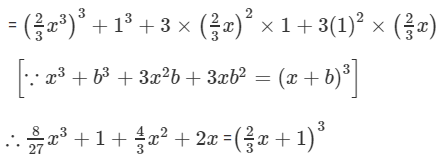Question 4: Factorize 8x3 + 27y3 + 36x2y + 54xy2

Solution:

8x3 + 27y3 + 36x2y + 54xy2

Above expression can be written as (2x)3 + (3y) 3 + 3×(2x)2×3y + 3×(2x)(3y)2

Which is similar to a³ + b³ + 3a²b + 3ab² = (a + b) ³]

Here a = 2x and b = 3y

= (2x+3y)3

Therefore, 8x3 + 27y3 + 36x2y + 54xy2 = (2x+3y)3

Question 5: Factorize a3 − 3a2b + 3ab2 − b3 + 8

Solution:

a3 − 3a2b + 3ab2 − b3 + 8

Using: a3 − b3 − 3a2b + 3ab2 = (a−b)3

= (a−b)3 + 23

Again , Using: a3 + b3 =(a + b)(a2 – ab + b2)]

=(a−b+2)((a−b)2−(a−b) × 2 + 22)

=(a−b+2)(a2+b2−2ab−2(a−b)+4)

=(a−b+2)(a2+b2−2ab−2a+2b+4)

a3 − 3a2b + 3ab2 − b3 + 8 =(a−b+2)(a2+b2−2ab−2a+2b+4)

### Exercise 5.4 Page No: 5.22

Factorize each of the following expressions:

Question 1: a3 + 8b3 + 64c3 − 24abc

Solution:

a3 + 8b3 + 64c3 − 24abc

= (a)3 + (2b) 3 + (4c) 3− 3×a×2b×4c

[Using a3+b3+c3−3abc = (a+b+c)(a2+b2+c2−ab−bc−ca)]

= (a+2b+4c)(a2+(2b)2 + (4c)2−a×2b−2b×4c−4c×a)

= (a+2b+4c)(a2 +4b2 +16c2 −2ab−8bc−4ac)

Therefore, a3 + 8b3 + 64c3 − 24abc = (a+2b+4c)(a2 +4b2 +16c2 −2ab−8bc−4ac)

Question 2: x 3 − 8y 3+ 27z3 + 18xyz

Solution:

= x3 − (2y) 3 + (3z) 3 − 3×x×(−2y)(3z)

= (x + (−2y) + 3z) (x2 + (−2y)2 + (3z) 2 −x(−2y)−(−2y)(3z)−3z(x))

[using a3+b3+c3−3abc = (a+b+c)(a2+b2+c2−ab−bc−ca)]

=(x −2y + 3z)(x2 + 4y2 + 9 z2 + 2xy + 6yz − 3zx)

Question 3: 27x 3 − y 3– z3 – 9xyz

Solution:

27x 3 − y 3– z3 – 9xyz

= (3x) 3 − y 3– z3 – 3(3xyz)

[Using a3 + b3 + c3 −3abc = (a + b + c)(a2+b2+c2−ab−bc−ca)]

Here a = 3x, b = -y and c = -z

= (3x – y – z){ (3x)2 + (- y)2 + (– z)2 + 3xy – yz + 3xz)}

= (3x – y – z){ 9x2 + y2 + z2 + 3xy – yz + 3xz)}

Question 4: 1/27 x3 − y3 + 125z3 + 5xyz

Solution:

1/27 x3 − y3 + 125z3 + 5xyz

= (x/3)3+(−y)3 +(5z)3 – 3 x/3 (−y)(5z)

[Using a3 + b3 + c3 −3abc = (a + b + c)(a2+b2+c2−ab−bc−ca)]

= (x/3 + (−y) + 5z)((x/3)2 + (−y)2 + (5z) 2 –x/3(−y) − (−y)5z−5z(x/3))

= (x/3 −y + 5z) (x^2/9 + y2 + 25z2 + xy/3 + 5yz – 5zx/3)

Question 5: 8x3 + 27y3 − 216z3 + 108xyz

Solution:

8x3 + 27y3 − 216z3 + 108xyz

= (2x) 3 + (3y) 3 +(−6y) 3 −3(2x)(3y)(−6z)

= (2x+3y+(−6z)){ (2x)2+(3y) 2+(−6z) 2 −2x×3y−3y(−6z)−(−6z)2x}

= (2x+3y−6z) {4x2 +9y2 +36z2 −6xy + 18yz + 12zx}

Question 6: 125 + 8x3 − 27y3 + 90xy

Solution:

125 + 8x3 − 27y3 + 90xy

= (5)3 + (2x) 3 +(−3y) 3 −3×5×2x×(−3y)

= (5+2x+(−3y)) (52 +(2x) 2 +(−3y) 2 −5(2x)−2x(−3y)−(−3y)5)

= (5+2x−3y)(25+4x2 +9y2 −10x+6xy+15y)

Question 7: (3x−2y)3 + (2y−4z) 3 + (4z−3x) 3

Solution:

(3x−2y)3 + (2y−4z) 3 + (4z−3x) 3

Let (3x−2y) = a, (2y−4z) = b , (4z−3x) = c

a + b + c= 3x−2y+2y−4z+4z−3x = 0

We know, a3 + b3 + c3 −3abc = (a + b + c)(a2+b2+c2−ab−bc−ca)

⇒ a3 + b3 + c3 −3abc = 0

or a3 + b3 + c3 =3abc

⇒ (3x−2y)3 + (2y−4z) 3 + (4z−3x) 3 = 3(3x−2y)(2y−4z)(4z−3x)

Question 8: (2x−3y)3 + (4z−2x) 3 + (3y−4z) 3

Solution:

(2x−3y)3 + (4z−2x) 3 + (3y−4z) 3

Let 2x – 3y = a , 4z – 2x = b , 3y – 4z = c

a + b + c= 2x – 3y + 4z – 2x + 3y – 4z = 0

We know, a3 + b3 + c3 −3abc = (a + b + c)(a2+b2+c2−ab−bc−ca)

⇒ a3 + b3 + c3 −3abc = 0

(2x−3y)3 + (4z−2x) 3 + (3y−4z) 3 = 3(2x−3y)(4z−2x)(3y−4z)

### Exercise VSAQs Page No: 5.24

Question 1: Factorize x4 + x2 + 25

Solution:

x4 + x2 + 25

= (x2) 2 + 52 + x2

[using a2 + b2 = (a + b) 2 – 2ab ]

= (x2 +5) 2 −2(x2 ) (5) + x2

=(x2 +5) 2 −10x2 + x2

=(x2 + 5) 2 − 9x2

=(x2 + 5) 2 − (3x) 2

[using a2 – b2 = (a + b)(a – b ]

= (x 2 + 3x + 5)(x2 − 3x + 5)

Question 2: Factorize x2 – 1 – 2a – a2

Solution:

x2 – 1 – 2a – a2

x2 – (1 + 2a + a2 )

x2 – (a + 1)2

(x – (a + 1)(x + (a + 1)

(x – a – 1)(x + a + 1)

[using a2 – b2 = (a + b)(a – b) and (a + b)^2 = a^2 + b^2 + 2ab ]

Question 3: If a + b + c =0, then write the value of a3 + b3 + c3.

Solution:

We know, a3 + b3 + c3 – 3abc = (a + b +c ) (a2 + b2 + c2 – ab – bc − ca)

Put a + b + c =0

This implies

a3 + b3 + c3 = 3abc

Question 4: If a2 + b2 + c2 = 20 and a + b + c =0, find ab + bc + ca.

Solution:

We know, (a+b+c)² = a² + b² + c² + 2(ab + bc + ca)

0 = 20 + 2(ab + bc + ca)

-10 = ab + bc + ca

Or ab + bc + ca = -10

Question 5: If a + b + c = 9 and ab + bc + ca = 40, find a2 + b2 + c2 .

Solution:

We know, (a+b+c)² = a² + b² + c² + 2(ab + bc + ca)

92 = a² + b² + c² + 2(40)

81 = a² + b² + c² + 80

⇒ a² + b² + c² = 1

### RD Sharma Solutions for Class 9 Maths Chapter 5 Factorization of Algebraic Expressions

In the 5th Chapter of Class 9 RD Sharma Solutions students will study important topics as listed below:

• Factorization by taking out the common factors
• Factorization by grouping the terms
• Factorization by making a perfect square
• Factorization by the difference of two squares
• Factorization of quadratic polynomials by splitting the middle term
• Factorization of algebraic expressions expressible as the sum or difference of two cubes

Students who want to gain proficiency in Mathematics  are advised to practice textbook questions as many times as possible using solutions provided at BYJU’S website. This also helps them to come out with flying colours in examinations.

## Frequently Asked Questions on RD Sharma Solutions for Class 9 Maths Chapter 5

### Mention the topics present in RD Sharma Solutions for Class 9 Maths Chapter 5.

The topics present in RD Sharma Solutions for Class 9 Maths Chapter 5 are as follows:,
1. Factorization by taking out the common factors
2. Factorization by grouping the terms
3. Factorization by making a perfect square
4. Factorization by the difference of two squares
5. Factorization of quadratic polynomials by splitting the middle term
6. Factorization of algebraic expressions expressible as the sum or difference of two cubes.

### Is it necessary to learn all the questions present in RD Sharma Solutions for Class 9 Maths Chapter 5?

Yes, students must practice the questions present in the Chapter 5 of RD Sharma Solutions Class 9 Maths to secure high marks in the exams. The solutions present in BYJU’S website are very accurate and clear. Students can make use of these solutions to clear their doubts immediately. These solutions help students in grasping the concepts effectively and also boosts confidence in solving the problems in a short period of time.

### Is RD Sharma Solutions for Class 9 Maths Chapter 5 difficult to understand?

No not at all, practising RD Sharma Solutions for Class 9 Maths Chapter 5 on a daily basis helps students to grasp the concepts easily. The main aim of these solutions is to provide a fundamental aspect of Maths, which in turn, helps the students to understand every concept in a descriptive manner. Following these solutions regularly also boosts student’s skills which are essential from an exam perspective.